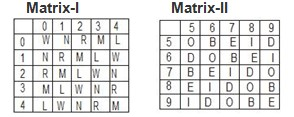# A word is represented by only one set of numbers as given in any of the alternatives. The sets of numbers given in the alternatives are represented by two classes of alphabets as in two matrices given below. The columns and rows of Matrix I are numbered from 0 to 4 and that of Matrix II are numbered from 5 to 9. A letter from these matrices can be represented first by its row and next by its column. e.g. L can be represented by 04, 31, 40 and B can be represented by 56, 98, 75 etc. Identify the set for the word WINNER.A 32, 86, 33, 24, 76, 43
B 32, 69, 22, 41, 57, 43
C 00, 59, 33, 10, 99, 98
D 23, 86, 32, 42, 76, 34

×
×